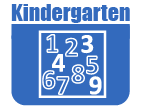# Math - Kindergarten

IntroductionIn kindergarten, instructional time should focus on two critical areas: (1) representing, relating, and operating on whole numbers, initially with sets of objects; (2) describing shapes and space. More learning time in kindergarten should be devoted to number than to other topics.

(1) Students will use numbers, including written numerals, to represent quantities and to solve quantitative problems, such as counting objects in a set; counting out a given number of objects; comparing sets or numerals; and modeling simple joining and separating situations with sets of objects, or eventually with equations such as 5 + 2 = 7 and 7 – 2 = 5. (Kindergarten students should see addition and subtraction equations, and student writing of equations in kindergarten is encouraged, but is not required.) Students will choose, combine, and apply effective strategies for answering quantitative questions, including quickly recognizing the cardinalities of small sets of objects, counting and producing sets of given sizes, counting the number of objects in combined sets, or counting the number of objects that remain in a set after some are taken away.

(2) Students will describe their physical world using geometric ideas (for example, shape, orientation, spatial relations) and vocabulary. They will identify, name, and describe basic two-dimensional shapes, such as squares, triangles, circles, rectangles, and hexagons, presented in a variety of ways (for example, with different sizes and orientations), as well as three-dimensional shapes such as cubes, cones, cylinders, and spheres. They will use basic shapes and spatial reasoning to model objects in their environment and to construct more complex shapes.The Online Core Resource pages are a collaborative project between the Utah State Board of Education and the Utah Education Network. If you would like to recommend a high quality resource, contact Trish French (Elementary) or Lindsey Henderson (Secondary). If you find inaccuracies or broken links contact resources@uen.org.